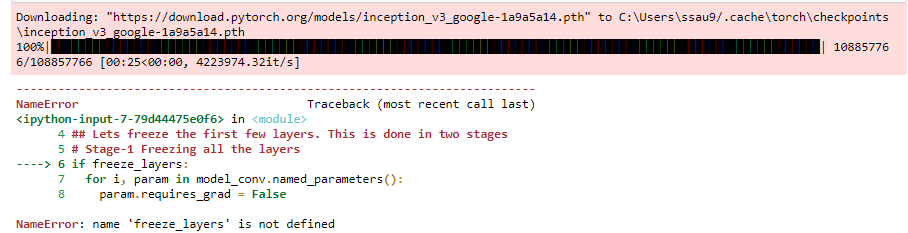{{ message }}

Instantly share code, notes, and snippets.

# L0SG/freeze_example.py

Last active Jul 29, 2021
PyTorch example: freezing a part of the net (including fine-tuning)
 import torch from torch import nn from torch.autograd import Variable import torch.nn.functional as F import torch.optim as optim # toy feed-forward net class Net(nn.Module): def __init__(self): super(Net, self).__init__() self.fc1 = nn.Linear(10, 5) self.fc2 = nn.Linear(5, 5) self.fc3 = nn.Linear(5, 1) def forward(self, x): x = self.fc1(x) x = self.fc2(x) x = self.fc3(x) return x # define random data random_input = Variable(torch.randn(10,)) random_target = Variable(torch.randn(1,)) # define net net = Net() # print fc2 weight print('fc2 weight before train:') print(net.fc2.weight) # train the net criterion = nn.MSELoss() optimizer = optim.SGD(net.parameters(), lr=0.1) for i in range(100): net.zero_grad() output = net(random_input) loss = criterion(output, random_target) loss.backward() optimizer.step() # print the trained fc2 weight print('fc2 weight after train:') print(net.fc2.weight) # save the net torch.save(net.state_dict(), 'model') # delete and redefine the net del net net = Net() # load the weight net.load_state_dict(torch.load('model')) # print the pre-trained fc2 weight print('fc2 pretrained weight (same as the one above):') print(net.fc2.weight) # define new random data random_input = Variable(torch.randn(10,)) random_target = Variable(torch.randn(1,)) # we want to freeze the fc2 layer this time: only train fc1 and fc3 net.fc2.weight.requires_grad = False net.fc2.bias.requires_grad = False # train again criterion = nn.MSELoss() # NOTE: pytorch optimizer explicitly accepts parameter that requires grad # see https://github.com/pytorch/pytorch/issues/679 optimizer = optim.Adam(filter(lambda p: p.requires_grad, net.parameters()), lr=0.1) # this raises ValueError: optimizing a parameter that doesn't require gradients #optimizer = optim.Adam(net.parameters(), lr=0.1) for i in range(100): net.zero_grad() output = net(random_input) loss = criterion(output, random_target) loss.backward() optimizer.step() # print the retrained fc2 weight # note that the weight is same as the one before retraining: only fc1 & fc3 changed print('fc2 weight (frozen) after retrain:') print(net.fc2.weight) # let's unfreeze the fc2 layer this time for extra tuning net.fc2.weight.requires_grad = True net.fc2.bias.requires_grad = True # add the unfrozen fc2 weight to the current optimizer optimizer.add_param_group({'params': net.fc2.parameters()}) # re-retrain for i in range(100): net.zero_grad() output = net(random_input) loss = criterion(output, random_target) loss.backward() optimizer.step() # print the re-retrained fc2 weight # note that this time the fc2 weight also changed print('fc2 weight (unfrozen) after re-retrain:') print(net.fc2.weight)

###codeeppy commented Jul 31, 2019

 Hey thanks for the code snippet! But there is an error while freezing the layer because it is not defined. Could you fix that and commit to the repository?###Sam-gege commented May 21, 2021

 Hi Thank you for you example! I'm a new learner, so just to make sure, it seems in the torch version I'm using ('1.8.1+cu102'), using "optimizer = optim.Adam(net.parameters(), lr=0.1)" no longer throws an error, and everything still works (fc2 doesn't change, fc1and fc3 changes) after unfreezing fc2, I don't need to write "optimizer.add_param_group({'params': net.fc2.parameters()})", the optimizer will automatically update parameters of fc2.